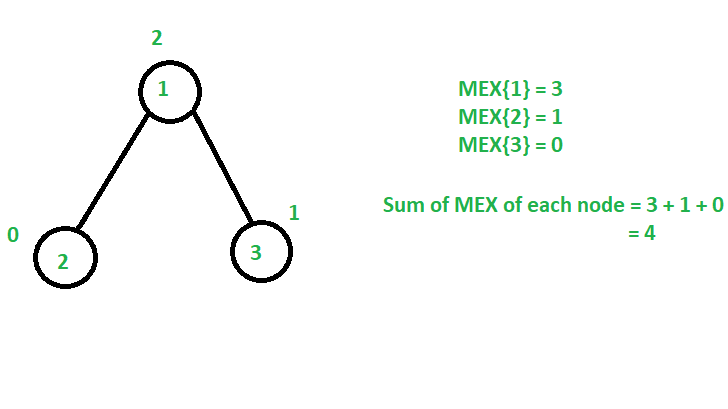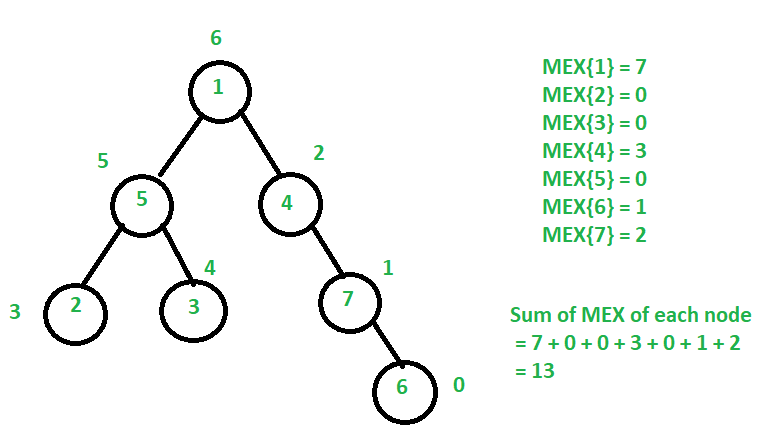Related Articles

# Maximize sum of MEX values of each node in an N-ary Tree

• Difficulty Level : Expert
• Last Updated : 18 May, 2021

Given an N-ary tree rooted at 1, the task is to assign values from the range [0, N – 1] to each node in any order such that the sum of MEX values of each node in the tree is maximized and print the maximum possible sum of MEX values of each node in the tree.

The MEX value of node V is defined as the smallest missing positive number in a tree rooted at node V.

Examples:

Input: N = 3, Edges[] = {{1, 2}, {1, 3}}
Output: 4
Explanation:Assign value 0 to node 2, 1 to node 3 and 2 to node 1.
Therefore, the maximum sum of MEX of all nodes = MEX{1} + MEX{2} + MEX{3} = 3 + 1 + 0 = 4.

Input: N = 7, Edges[] = {1, 5}, {1, 4}, {5, 2}, {5, 3}, {4, 7}, {7, 6}}
Output: 13
Explanation:Assign value 0 to node 6, 1 to node 7, 2 to node 4, 6 to node 1, 5 to node 5, 3 to node 2 and 4 to node 3.
Therefore, the maximum sum of MEX of all nodes = MEX{1} + MEX{2} + MEX{3} + MEX{4} + MEX{5} + MEX{6} + MEX{7} = 7 + 0 + 0 + 3 + 0 + 1 + 0 = 13.

Approach: The idea is to perform DFS Traversal on the given N-ary tree and find the sum of MEX for each subtree in the tree. Follow the steps below to solve the problem:

• Perform Depth First Search(DFS) on tree rooted at node 1.
• Initialize a variable mex with 0 and size with 1.
• Iterate through all children of the current node and perform the following operations:
• Recursively call the children of the current node and store the maximum sum of MEX among all subtree in mex.
• Increase the size of the tree rooted at the current node.
• Increase the value of mex by size.
• After completing the above steps, print the value of mex as the answer.

Below is the implementation of the above approach:

## C++

 `// C++ program for the above approach``#include ``using` `namespace` `std;` `// Function to create an N-ary Tree``void` `makeTree(vector<``int``> tree[],``              ``pair<``int``, ``int``> edges[],``              ``int` `N)``{``    ``// Traverse the edges``    ``for` `(``int` `i = 0; i < N - 1; i++) {` `        ``int` `u = edges[i].first;``        ``int` `v = edges[i].second;` `        ``// Add edges``        ``tree[u].push_back(v);``    ``}``}` `// Function to get the maximum sum``// of MEX values of tree rooted at 1``pair<``int``, ``int``> dfs(``int` `node,``                   ``vector<``int``> tree[])``{``    ``// Initialize mex``    ``int` `mex = 0;` `    ``int` `size = 1;` `    ``// Iterate through all children``    ``// of node``    ``for` `(``int` `u : tree[node]) {` `        ``// Recursively find maximum sum``        ``// of MEX values of each node``        ``// in tree rooted at u``        ``pair<``int``, ``int``> temp = dfs(u, tree);` `        ``// Store the maximum sum of MEX``        ``// of among all subtrees``        ``mex = max(mex, temp.first);` `        ``// Increase the size of tree``        ``// rooted at current node``        ``size += temp.second;``    ``}` `    ``// Resulting MEX for the current``    ``// node of the recursive call``    ``return` `{ mex + size, size };``}` `// Driver Code``int` `main()``{``    ``// Given N nodes``    ``int` `N = 7;` `    ``// Given N-1 edges``    ``pair<``int``, ``int``> edges[]``        ``= { { 1, 4 }, { 1, 5 }, { 5, 2 }, { 5, 3 }, { 4, 7 }, { 7, 6 } };` `    ``// Stores the tree``    ``vector<``int``> tree[N + 1];` `    ``// Generates the tree``    ``makeTree(tree, edges, N);` `    ``// Returns maximum sum of MEX``    ``// values of each node``    ``cout << dfs(1, tree).first;` `    ``return` `0;``}`

## Java

 `// Java program for the above approach``import` `java.util.*;` `class` `GFG{` `static` `class` `pair``{``    ``int` `first, second;``    ` `    ``public` `pair(``int` `first, ``int` `second)``    ``{``        ``this``.first = first;``        ``this``.second = second;``    ``}``}` `// Function to create an N-ary Tree``static` `void` `makeTree(Vector tree[],``                     ``pair edges[], ``int` `N)``{``    ` `    ``// Traverse the edges``    ``for``(``int` `i = ``0``; i < N - ``1``; i++)``    ``{``        ``int` `u = edges[i].first;``        ``int` `v = edges[i].second;``        ` `        ``// Add edges``        ``tree[u].add(v);``    ``}``}` `// Function to get the maximum sum``// of MEX values of tree rooted at 1``static` `pair dfs(``int` `node, Vector tree[])``{``    ` `    ``// Initialize mex``    ``int` `mex = ``0``;` `    ``int` `size = ``1``;` `    ``// Iterate through all children``    ``// of node``    ``for``(``int` `u : tree[node])``    ``{``        ` `        ``// Recursively find maximum sum``        ``// of MEX values of each node``        ``// in tree rooted at u``        ``pair temp = dfs(u, tree);``        ` `        ``// Store the maximum sum of MEX``        ``// of among all subtrees``        ``mex = Math.max(mex, temp.first);``        ` `        ``// Increase the size of tree``        ``// rooted at current node``        ``size += temp.second;``    ``}``    ` `    ``// Resulting MEX for the current``    ``// node of the recursive call``    ``return` `new` `pair(mex + size, size);``}` `// Driver Code``public` `static` `void` `main(String[] args)``{``    ` `    ``// Given N nodes``    ``int` `N = ``7``;` `    ``// Given N-1 edges``    ``pair edges[] = { ``new` `pair(``1``, ``4``),``                     ``new` `pair(``1``, ``5``),``                     ``new` `pair(``5``, ``2``),``                     ``new` `pair(``5``, ``3``),``                     ``new` `pair(``4``, ``7``),``                     ``new` `pair(``7``, ``6``) };` `    ``// Stores the tree``    ``@SuppressWarnings``(``"unchecked"``)``    ``Vector[] tree = ``new` `Vector[N + ``1``];``    ``for``(``int` `i = ``0``; i < tree.length; i++)``        ``tree[i] = ``new` `Vector();``        ` `    ``// Generates the tree``    ``makeTree(tree, edges, N);` `    ``// Returns maximum sum of MEX``    ``// values of each node``    ``System.out.print((dfs(``1``, tree).first));``}``}` `// This code is contributed by Princi Singh`

## Python3

 `# Python3 program for the above approach` `# Function to create an N-ary Tree``def` `makeTree(tree, edges, N):``    ` `    ``# Traverse the edges``    ``for` `i ``in` `range``(N ``-` `1``):``        ``u ``=` `edges[i][``0``]``        ``v ``=` `edges[i][``1``]` `        ``# Add edges``        ``tree[u].append(v)``        ` `    ``return` `tree` `# Function to get the maximum sum``# of MEX values of tree rooted at 1``def` `dfs(node, tree):``    ` `    ``# Initialize mex``    ``mex ``=` `0` `    ``size ``=` `1` `    ``# Iterate through all children``    ``# of node``    ``for` `u ``in` `tree[node]:` `        ``# Recursively find maximum sum``        ``# of MEX values of each node``        ``# in tree rooted at u``        ``temp ``=` `dfs(u, tree)` `        ``# Store the maximum sum of MEX``        ``# of among all subtrees``        ``mex ``=` `max``(mex, temp[``0``])` `        ``# Increase the size of tree``        ``# rooted at current node``        ``size ``+``=` `temp[``1``]` `    ``# Resulting MEX for the current``    ``# node of the recursive call``    ``return` `[mex ``+` `size, size]` `# Driver Code``if` `__name__ ``=``=` `'__main__'``:``    ` `    ``# Given N nodes``    ``N ``=` `7` `    ``# Given N-1 edges``    ``edges ``=` `[ [ ``1``, ``4` `], [ ``1``, ``5` `],``              ``[ ``5``, ``2` `], [ ``5``, ``3` `],``              ``[ ``4``, ``7` `], [ ``7``, ``6` `] ]` `    ``# Stores the tree``    ``tree ``=` `[[] ``for` `i ``in` `range``(N ``+` `1``)]` `    ``# Generates the tree``    ``tree ``=` `makeTree(tree, edges, N)` `    ``# Returns maximum sum of MEX``    ``# values of each node``    ``print``(dfs(``1``, tree)[``0``])` `# This code is contributed by mohit kumar 29`

## C#

 `// C# program for the above approach``using` `System;``using` `System.Collections.Generic;` `class` `GFG{` `public` `class` `pair``{``    ``public` `int` `first, second;``    ` `    ``public` `pair(``int` `first, ``int` `second)``    ``{``        ``this``.first = first;``        ``this``.second = second;``    ``}``}` `// Function to create an N-ary Tree``static` `void` `makeTree(List<``int``> []tree,``                     ``pair []edges, ``int` `N)``{``    ` `    ``// Traverse the edges``    ``for``(``int` `i = 0; i < N - 1; i++)``    ``{``        ``int` `u = edges[i].first;``        ``int` `v = edges[i].second;``        ` `        ``// Add edges``        ``tree[u].Add(v);``    ``}``}` `// Function to get the maximum sum``// of MEX values of tree rooted at 1``static` `pair dfs(``int` `node, List<``int``> []tree)``{``    ` `    ``// Initialize mex``    ``int` `mex = 0;``    ` `    ``int` `size = 1;``    ` `    ``// Iterate through all children``    ``// of node``    ``foreach``(``int` `u ``in` `tree[node])``    ``{``        ` `        ``// Recursively find maximum sum``        ``// of MEX values of each node``        ``// in tree rooted at u``        ``pair temp = dfs(u, tree);``        ` `        ``// Store the maximum sum of MEX``        ``// of among all subtrees``        ``mex = Math.Max(mex, temp.first);``        ` `        ``// Increase the size of tree``        ``// rooted at current node``        ``size += temp.second;``    ``}``    ` `    ``// Resulting MEX for the current``    ``// node of the recursive call``    ``return` `new` `pair(mex + size, size);``}` `// Driver Code``public` `static` `void` `Main(String[] args)``{``    ` `    ``// Given N nodes``    ``int` `N = 7;``    ` `    ``// Given N-1 edges``    ``pair []edges = { ``new` `pair(1, 4),``                     ``new` `pair(1, 5),``                     ``new` `pair(5, 2),``                     ``new` `pair(5, 3),``                     ``new` `pair(4, 7),``                     ``new` `pair(7, 6) };` `    ``// Stores the tree``    ``List<``int``>[] tree = ``new` `List<``int``>[N + 1];``    ``for``(``int` `i = 0; i < tree.Length; i++)``        ``tree[i] = ``new` `List<``int``>();``        ` `    ``// Generates the tree``    ``makeTree(tree, edges, N);``    ` `    ``// Returns maximum sum of MEX``    ``// values of each node``    ``Console.Write((dfs(1, tree).first));``}``}` `// This code is contributed by Amit Katiyar`

## Javascript

 ``
Output:
`13`

Time Complexity: O(N)
Auxiliary Space: O(1)

Attention reader! Don’t stop learning now. Get hold of all the important DSA concepts with the DSA Self Paced Course at a student-friendly price and become industry ready.  To complete your preparation from learning a language to DS Algo and many more,  please refer Complete Interview Preparation Course.

In case you wish to attend live classes with experts, please refer DSA Live Classes for Working Professionals and Competitive Programming Live for Students.

My Personal Notes arrow_drop_up